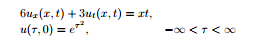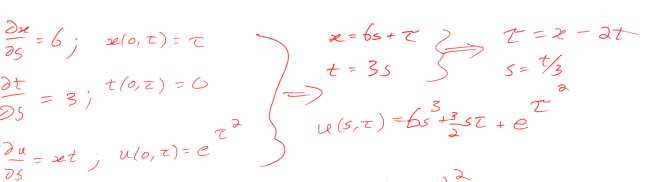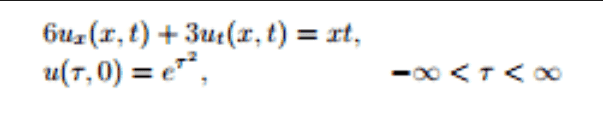# Missing Power Solving the PDE (Solution included)

• zr95

## Homework Statement## The Attempt at a Solution

integral[du]= Integral[xt ds]
xt=18s2+3sT
so,
u=Integral[18s2+3sT]
u=6s3+(3/2)s2T+C
C=eT2

This is what I did and the solution is below. I'm unsure where the missing power on the (3/2)sT went in the u(s,t) equation.[/B]## Homework Statement

View attachment 100094

## The Attempt at a Solution

integral[du]= Integral[xt ds]
xt=18s2+3sT
so,
u=Integral[18s2+3sT]
u=6s3+(3/2)s2T+C
C=eT2

This is what I did and the solution is below. I'm unsure where the missing power on the (3/2)sT went in the u(s,t) equation.
View attachment 100096
Let's make the initial image somewhat readable:You reaaly need to give a complete statement of your problem in the body of your initial post. Here, are we to assume that you are asked to solve that PDE with the stated initial condition?

The goal is to solve the cauchy pde.